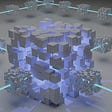The red black tree, is bit difficult algorithm (at least for me) to understand at the first glance. Initially personally thought of writing this article in my mother language but to be honest Japanese is pretty complex (inefficient) language to express (because i need to switch to and fro between alphabet and kanas, plus kana IME is pretty stupid such that I feel like smashing my corporate PC with hammer so…).

Okay, the reason why red-black tree exists, aside from the binary search tree is for the tree data set of which its hight h is pretty high, then the runtime of binary search tree is not that fast. (O(n) ? correct me if i’m wrong), meanwhile the red black tree is much faster (O(lgn) time for any actions).

The red black trees are different from binary search ones for it has the following properties:

color(red | black), key, left, right, and p (parent)

It has the following rules:

1. every node must be either red or black.

2. the root node must be black.

3. every leaf(NIL) is black.

4. if a node is red, then the both its children are black.

5. The same layer (height) of node should have the same amount of black nodes.

(Referenced from mit press Introduction to algorithms.)

The feature of this tree structure is sentinel, since it ends with NIL, plus in order to save memory space, all the leaves (NIL) are deducted to a single NIL node.

Okay, then let me explain about the rotation:

there is left_rotate and right_rotate.

And left_rotate and right_rotate makes to transform the tree into the counter part.

Yet can you directly understand how it works? Since I am not that smart guy as you, I’m not.

So I decided to find two patterns here.

The blue line is TAKE-LINE (blue: stupid name I personally created so you don’t need to remember this) and GARBAGE (yellow) node.

TAKE LINE is one single line which connects Y and A (or C) and the line is one straight line, not twisted.

Then, forget about the top node of the TAKE LINE and just focus on the bottom two (on the left Y, C and on the right X, A).

You take this (left)X-A or (right)Y-C with you.

On the other hand, the yellow GARBAGE node is the miserable node which regarded by its parent node as garbage, so it is split (unfortunately) from its parent node and given to the counter parts.

Then the idea which child node is going to be given to the parent node and which is taken is clear.

Then, let’s look at the pseudo code given by the Introduction to Algorithms:

Let me go through this code.

x.right = y. left …. (1)

y want to give its GARBAGE (y.left = B node) to x. x already has its kid on its left A such that x can only accept the new child to its right. So y gives its unwanted GARBAGE to the y’s right.

if y.left != T.nil

y.left.p = x …. (2)

This means the same thing to (1), just this is reiteration of (1), then why this is needed? It is because this is GARBAGE’s view (B node).

The (1) says,

I am B’s parent. I don’t want B (= GARBAGE) so that I give it to the other guy (x).

The (2) says,

I am B. If I exist, my new papa is x. 😂

So this means the same thing. Yet, as the other linked-based structures, (such as linked-list) the same relation ship should be stated in both A and B. For instance, if A is the father of B, then A need to say “I’m A, B is my son.” And B need to say “I’m B, A is my daddy” as well. Neither of these statements should be absent, this is what is going on in (1) and (2).

Okay, then

y.p = x.p

says about the R. since y is going to be the parent of the x now,

R-x-y…

must be changed to the

R-y-x…

To say it in one word,

R-x-y.. → R-y-x..

In other words,

[x.p]-x-y..→ [y.p]-y-x..

pretty obvious.

Yet as I stated earlier, this is the viewpoint of only x and y. x gave up saying his father is R, and y now proclaims its dad is R.

Now it’s R’s turn:

if x.p == T.nil … (3)

T.root = y

elsif x == x.p.left … (4)

x.p.left = y

else x.p.right = y … (5)

If there is no R, then y is the top of the hierarchy. … (3)

Or, if the x used to be the left of the R, then y should be located at the left of the R.

Or, if the x used to be the right of the R, then y should be located at the right of the R.

Finally, we need to define on which direction x (as kid of y) should be located under the y, and since y already TAKEN C to it’s right, so the only left of the y is available,

y.left = x … (6)

and since in (6) y has admitted that its left child is x, then it’s x’s turn to admit that its daddy is now y.

x.p = y

Something like this.

Yeah since the algorithm around here is hard to get at the first glance, but if you dig deeper, maybe sometimes you can be enlightened.

--

--

--

## More from Kei Sugano

Love podcasts or audiobooks? Learn on the go with our new app.

## Recommended from Medium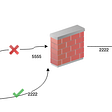## Git Troubleshooting — I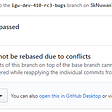## Free audits are here## Learning Path For Second Year CSE Undergraduate Students📚💻## Managing Zowe’s App Server (and ZSS) via its Admin API## OpenStack Overcloud Architecture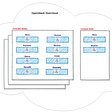## Les Embiez 2021 : Digital Edition## My Week-3 at JADU a Full Stack Development Program.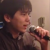## Binary Search(4) / Algorithm## 563. Binary Tree Tilt 🚀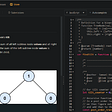## Solution to the k-closest point in space problem